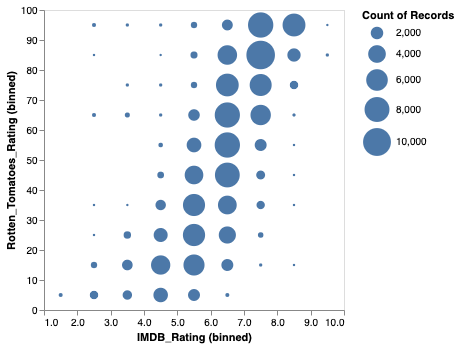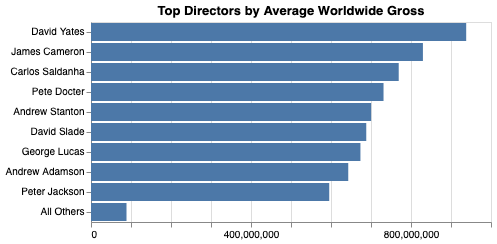# Polars

Polars describes itself as a “Lightning-fast DataFrame library for Rust and Python”. Polars has quickly gained popularity as a faster alternative to pandas that also supports larger datasets.

VegaFusion’s Polars integration makes it possible to input and output Polars DataFrames without conversion through pandas.

Note: Polars support requires Altair 5.

## Polars as Input

Here is a full example that uses Polars to read a 201k row movies dataset from a remote parquet file. The DataFrame’s `filter` method is then used to remove rows with NULL movie rating values. This filtered DataFrame is passed as the input to an Altair chart.

```import polars as pl
import vegafusion as vf
import altair as alt

# Enable Mime Renderer
vf.enable()

# Load 201k movies parquet dataset
"https://vegafusion-datasets.s3.amazonaws.com/vega/movies_201k.parquet"
)

# Fitler out rows with null ratings
source = source.filter(
pl.col("IMDB_Rating").is_not_null() & pl.col("Rotten_Tomatoes_Rating").is_not_null()
)

# Create chart
chart = alt.Chart(source).mark_circle().encode(
alt.X('IMDB_Rating:Q', bin=True),
alt.Y('Rotten_Tomatoes_Rating:Q', bin=True),
size='count()'
)
chart
```When a Polars DataFrame is provided as the input to a chart, it is loaded into the DataFusion (or DuckDB) query engine through the DataFrame Interchange Protocol.

## Polars as Output

When a Chart that references a Polars DataFrame is passed to `vegafusion.transformed_data`, the result will also be a Polars DataFrame. Here is a Polars version of the example from the Transformed Data section.

```import altair as alt
import vegafusion as vf
import polars a pl

# Enable mime renderer
vf.enable()

# Load 201k movies parquet dataset with Polars
"https://vegafusion-datasets.s3.amazonaws.com/vega/movies_201k.parquet"
)

# Build chart
chart = (
alt.Chart(source)
.transform_aggregate(
aggregate_gross='mean(Worldwide_Gross)',
groupby=["Director"],
).transform_window(
rank='row_number()',
sort=[alt.SortField("aggregate_gross", order="descending")],
).transform_calculate(
ranked_director="datum.rank < 10 ? datum.Director : 'All Others'"
).mark_bar().encode(
x=alt.X("aggregate_gross:Q", aggregate="mean", title=None),
y=alt.Y(
"ranked_director:N",
sort=alt.Sort(op="mean", field="aggregate_gross", order="descending"),
title=None,
),
)
).properties(
title="Top Directors by Average Worldwide Gross",
)
chart
```Now retrieve the chart’s transformed data as a Polars DataFrame.

```transformed = vf.transformed_data(chart)
print(type(transformed))
transformed
```
```<class 'polars.internals.dataframe.frame.DataFrame'>
```

shape: (10, 2)

ranked_director (str)

mean_aggregate_gross (f64)

David Yates

9.37984e+08

James Cameron

8.29781e+08

Carlos Saldanha

7.69293e+08

Pete Docter

7.31305e+08

Andrew Stanton

7.00319e+08

6.88155e+08

George Lucas

6.73577e+08